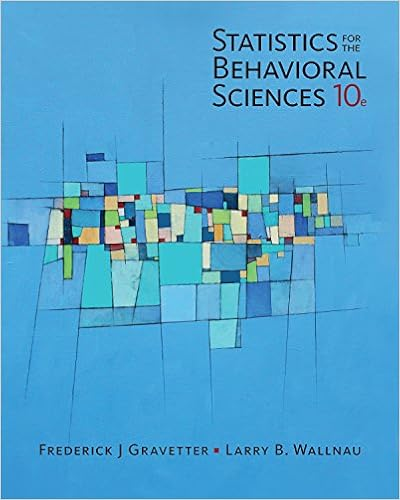# Referring to what is the value of the test statistic

• Notes
• PrivateBraveryWalrus9433
• 38
• 96% (202) 193 out of 202 people found this document helpful

This preview shows page 33 - 35 out of 38 pages.

##### We have textbook solutions for you!
The document you are viewing contains questions related to this textbook.The document you are viewing contains questions related to this textbook.
Chapter 4 / Exercise 2
Statistics for the Behavioral Sciences
Gravetter/WallnauExpert Verified
144.Referring to Table 10-11, what is the value of the test statistic to use in evaluating the alternative hypothesis that there is a difference in the two population proportions?a)4.335b)1.96c)1.093d)0ANSWER:c
TYPE: MC DIFFICULTY: ModerateKEYWORDS: Ztest, difference between two proportions, test statistic145.Referring to Table 10-11, the company tests to determine at the 0.05 level whether the population proportion has changed from the previous study. Which of the following is most correct?
##### We have textbook solutions for you!
The document you are viewing contains questions related to this textbook.The document you are viewing contains questions related to this textbook.
Chapter 4 / Exercise 2
Statistics for the Behavioral Sciences
Gravetter/WallnauExpert Verified
76 Two-Sample TestsbTYPE: MC DIFFICULTY: ModerateKEYWORDS: Ztest, difference between two proportions, decision, conclusion146.Referring to Table 10-11, construct a 99% confidence interval estimate of the difference in proportion of workers who would like to attend a self-improvement course in the recent study and the past study.
147.Referring to Table 10-11, construct a 95% confidence interval estimate of the difference in proportion of workers who would like to attend a self-improvement course in the recent study and the past study.
148.Referring to Table 10-11, construct a 90% confidence interval estimate of the difference in proportion of workers who would like to attend a self-improvement course in the recent study and the past study.
TYPE: PR DIFFICULTY: ModerateKEYWORDS: confidence interval, difference between two proportions149.True or False: In testing the difference between two proportions using the normal distribution, we may use a two-tailed Z test.
TYPE: TF DIFFICULTY: Easy KEYWORDS: Ztest, difference between two proportions150.If we wish to determine whether there is evidence that the proportion of successes is higher in Group 1 than in Group 2, and the test statistic for Z= +2.07 where the difference is defined as Group 1’s proportion minus Group 2’s proportion, the p-value is equal to ______.
TYPE: FI DIFFICULTY: Moderate KEYWORDS: Ztest, difference between two proportions, p-value151.If we wish to determine whether there is evidence that the proportion of successes is higher in Group 1 than in Group 2, and the test statistic for Z= -2.07 where the difference is defined as Group 1’s proportion minus Group 2’s proportion, the p-value is equal to ______.
•••